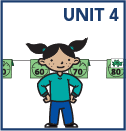## Grade 1

In grade 1 math, your child will:

• solve addition and subtraction story problems

• count by ones and tens to 120 read and write numbers to 120, and represent a number of objects up to 120 with a written numeral

• understand place value by describing what the digits mean in two-digit numbers; use this knowledge to add and subtract

• add two-digit numbers using at least two different strategies and explain how the strategies work

• subtract two-digit numbers that are multiples of 10 using at least two different strategies and explain how the strategies work

• compare two numbers using the symbols >, =, and <

• add and subtract numbers within 10 efficiently and accurately

• read or construct a graph and answer questions about the data

• measure length using non-standard units such as Popsicle sticks, linking cubes, and so on

• tell and write time to the hour and half-hour on analog and digital clock